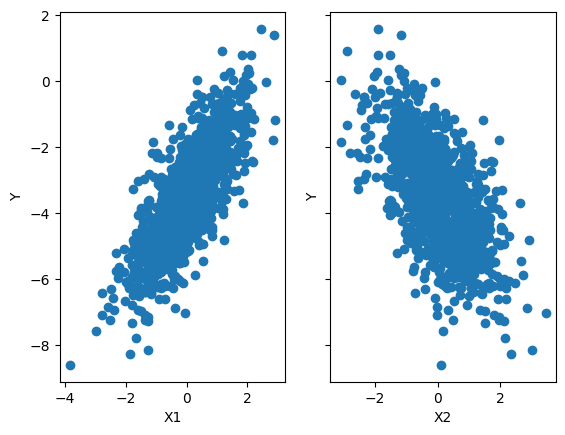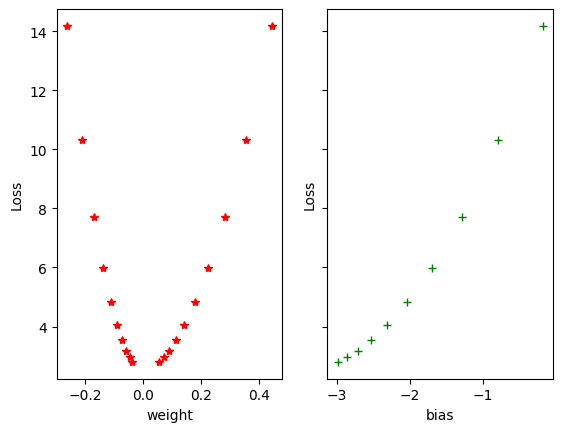Open In App

# Gradient Descent algorithm and its variants

Think about how a machine learns from the data in machine learning and deep learning during training.  This involves a large amount of data. In the case of supervised learning, this will be labeled data i.e input data paired with their respective label or target value. After the learning machine will be able to produce the correct outputs from similar input data i.e fed up before.

Deep learning is able to learn the complex representations of data when input and desired output are far from each other like image data and sequential data that comes from human languages & texts. Deep learning can learn even when human minds, traditional machine learning, and statistical approach fails to find the clue or even give very bad accuracy.  The learning happens during the backpropagation while training the neural network-based model. There is a term known as Gradient Descent, which is used to optimize the weight and biases based on the cost function. cost function evaluates the difference between the actual and predicted outputs.

Let’s understand  with an example:

## Python3

 import torchimport torch.nn as nnimport matplotlib.pyplot as plt

## Python3

 # set random seed for reproducibilitytorch.manual_seed(42) # set number of samplesnum_samples = 1000 # create random features with 2 dimensionsx = torch.randn(num_samples, 2) # create random weights and bias for the linear regression modeltrue_weights = torch.tensor([1.3, -1])true_bias    = torch.tensor([-3.5]) # Target variabley = x @ true_weights.T + true_bias # Plot the datasetfig, ax = plt.subplots(1, 2, sharey=True)ax.scatter(x[:,0],y)ax.scatter(x[:,1],y) ax.set_xlabel('X1')ax.set_ylabel('Y')ax.set_xlabel('X2')ax.set_ylabel('Y')plt.show()

Output:X vs Y

#### Let’s first try with a linear model:## Python3

 # Define the modelclass LinearRegression(nn.Module):    def __init__(self, input_size, output_size):        super(LinearRegression, self).__init__()        self.linear = nn.Linear(input_size, output_size)     def forward(self, x):        out = self.linear(x)        return out  # Define the input and output dimensionsinput_size = x.shapeoutput_size = 1 # Instantiate the modelmodel = LinearRegression(input_size, output_size)

#### Note:

The number of weight values will be equal to the input size of the model, And the input size in deep Learning is the number of independent input features i.e we are putting inside the model

In our case, input features are two so, the input size will also be two, and the corresponding weight value will also be two.

## Python3

 # create a random weight & bias tensorweight = torch.randn(1, input_size)bias   = torch.rand(1) # create a nn.Parameter object from the weight & bias tensorweight_param = nn.Parameter(weight)bias_param   = nn.Parameter(bias) # assign the weight & bias parameter to the linear layermodel.linear.weight = weight_parammodel.linear.bias   = bias_param weight, bias = model.parameters()print('Weight :',weight)print('bias :',bias)

Output:

Weight : Parameter containing:
bias : Parameter containing:
tensor([0.5710], requires_grad=True)

## Python3

 y_p = model(x)y_p[:5]

Output:

tensor([[ 0.7760],
[-0.8944],
[-0.3369],
[-0.3095],
[ 1.7338]], grad_fn=<SliceBackward0>)

#### Define the loss functionHere we are calculating the Mean Squared Error by taking the square of the difference between the actual and the predicted value and then dividing it by its length (i.e n = the Total number of output or target values) which is the mean of squared errors.

## Python3

 # Define the loss functiondef Mean_Squared_Error(prediction, actual):    error = (actual-prediction)**2    return error.mean()  # Find the total mean squared errorloss = Mean_Squared_Error(y_p, y)loss

Output:

tensor(19.9126, grad_fn=<MeanBackward0>)

As we can see from the above right now the Mean Squared Error is 30559.4473. All the steps which are done till now are known as forward propagation.

Now our task is to find the optimal value of weight w and bias b which can fit our model well by giving very less or minimum error as possible. i.eNow to update the weight and bias value and find the optimal value of weight and bias we will do backpropagation. Here the Gradient Descent comes into the role to find the optimal value weight and bias.

A gradient is nothing but a derivative that defines the effects on outputs of the function with a little bit of variation in inputs.

Gradient Descent (GD) is a widely used optimization algorithm in deep learning that is used to minimize the cost function of a neural network model during training. It works by iteratively adjusting the weights or parameters of the model in the direction of the negative gradient of the cost function until the minimum of the cost function is reached.

### How the Gradient Descent algorithm works:

For the sake of complexity, we can write our loss function for the single row as belowIn the above function x and y are our input data i.e constant. To find the optimal value of weight w and bias b. we partially differentiate with respect to w and b. This is also said that we will find the gradient of loss function J(w,b) with respect to w and b to find the optimal value of w and b.

#### Gradient of J(w,b) with respect to wi.e#### Gradient of J(w,b) with respect to bi.eHere we have considered the linear regression. So that here the parameters are weight and bias only. But in a fully connected neural network model there can be multiple layers and multiple parameters.  but the concept will be the same everywhere. And the below-mentioned formula will work everywhere.Here,

•= Learning rate
• J = Loss function
•= Gradient symbol denotes the derivative of loss function J
• Param = weight and bias     There can be multiple weight and bias values depending upon the complexity of the model and features in the dataset

In our case:In the current problem, two input features, So, the weight will be two.

### Implementations of the Gradient Descent algorithm for the above model

Steps:

1.  Find the gradient using loss.backward()
2. Get the parameter using model.linear.weight and model.linear.bias
3. Update the parameter using the above-defined equation.
4. Again assign the model parameter to our model
# Find the fradient using
loss.backward()

# Learning Rate
learning_rate = 0.001

# Model Parameter
w = model.linear.weight
b = model.linear.bias

# Matually Update the model parameter
w = w - learning_rate * w.grad
b = b - learning_rate * b.grad

# assign the weight & bias parameter to the linear layer
model.linear.weight = nn.Parameter(w)
model.linear.bias   = nn.Parameter(b)

## Python3

 # Number of epochsnum_epochs = 1000 # Learning Ratelearning_rate = 0.01 # SUBPLOT WEIGHT & BIAS VS lOSSESfig, (ax1, ax2) = plt.subplots(1, 2, sharey=True) for epoch in range(num_epochs):    # Forward pass    y_p = model(x)    loss = Mean_Squared_Error(y_p, y)         # Backproogation    # Find the fradient using    loss.backward()     # Learning Rate    learning_rate = 0.001     # Model Parameter    w = model.linear.weight    b = model.linear.bias     # Matually Update the model parameter    w = w - learning_rate * w.grad    b = b - learning_rate * b.grad     # assign the weight & bias parameter to the linear layer    model.linear.weight = nn.Parameter(w)    model.linear.bias   = nn.Parameter(b)                 if (epoch+1) % 100 == 0:        ax1.plot(w.detach().numpy(),loss.item(),'r*-')        ax2.plot(b.detach().numpy(),loss.item(),'g+-')        print('Epoch [{}/{}], weight:{}, bias:{} Loss: {:.4f}'.format(            epoch+1,num_epochs,            w.detach().numpy(),            b.detach().numpy(),            loss.item()))         ax1.set_xlabel('weight')ax2.set_xlabel('bias')ax1.set_ylabel('Loss')ax2.set_ylabel('Loss')plt.show()

Output:

Epoch [100/1000], weight:[[-0.2618025   0.44433367]], bias:[-0.17722966] Loss: 14.1803
Epoch [200/1000], weight:[[-0.21144074  0.35393423]], bias:[-0.7892358] Loss: 10.3030
Epoch [300/1000], weight:[[-0.17063744  0.28172654]], bias:[-1.2897989] Loss: 7.7120
Epoch [400/1000], weight:[[-0.13759881  0.22408141]], bias:[-1.699218] Loss: 5.9806
Epoch [500/1000], weight:[[-0.11086453  0.17808875]], bias:[-2.0340943] Loss: 4.8235
Epoch [600/1000], weight:[[-0.08924612  0.14141548]], bias:[-2.3080034] Loss: 4.0502
Epoch [700/1000], weight:[[-0.0717768   0.11219224]], bias:[-2.5320508] Loss: 3.5333
Epoch [800/1000], weight:[[-0.0576706   0.08892148]], bias:[-2.7153134] Loss: 3.1878
Epoch [900/1000], weight:[[-0.04628877  0.07040432]], bias:[-2.8652208] Loss: 2.9569
Epoch [1000/1000], weight:[[-0.0371125   0.05568104]], bias:[-2.9878428] Loss: 2.8026Weight & Bias vs Losses – Geeksforgeeks

From the above graph and data, we can observe the Losses are decreasing as per the weight and bias variations.

Now we have found the optimal weight and bias values. Print the optimal weight and bias and

## Python3

 w = model.linear.weightb = model.linear.bias print('weight(W) = {} \n  bias(b) = {}'.format(  w.abs(),  b.abs()))

Output:

weight(W) = tensor([[0.0371, 0.0557]], grad_fn=<AbsBackward0>)
bias(b) = tensor([2.9878], grad_fn=<AbsBackward0>)

## Python3

 pred =  x @ w.T + bpred[:5]

Output:

tensor([[-2.9765],
[-3.1385],
[-3.0818],
[-3.0756],
[-2.8681]], grad_fn=<SliceBackward0>)

Vanishing and exploding gradients are common problems that can occur during the training of deep neural networks. These problems can significantly slow down the training process or even prevent the network from learning altogether.

The vanishing gradient problem occurs when gradients become too small during backpropagation. The weights of the network are not considerably changed as a result, and the network is unable to discover the underlying patterns in the data. Many-layered deep neural networks are especially prone to this issue. The gradient values fall exponentially as they move backward through the layers, making it challenging to efficiently update the weights in the earlier layers.

The exploding gradient problem, on the other hand, occurs when gradients become too large during backpropagation. When this happens, the weights are updated by a large amount, which can cause the network to diverge or oscillate, making it difficult to converge to a good solution.

#### To address these problems the following technique can be used:

• Weights Regularzations: The initialization of weights can be adjusted to ensure that they are in an appropriate range. Using a different activation function, such as the Rectified Linear Unit (ReLU), can also help to mitigate the vanishing gradient problem.
• Gradient clipping: It involves limiting the maximum and minimum values of the gradient during backpropagation. This can prevent the gradients from becoming too large or too small and can help to stabilize the training process.
• Batch normalization: It can also help to address these problems by normalizing the input to each layer, which can prevent the activation function from saturating and help to reduce the vanishing and exploding gradient problems.

## Different Variants of Gradient Descent

There are several variants of gradient descent that differ in the way the step size or learning rate is chosen and the way the updates are made. Here are some popular variants:

1. Batch Gradient Descent: In batch gradient descent, To update the model parameter values like weight and bias, the entire training dataset is used to compute the gradient and update the parameters at each iteration. This can be slow for large datasets but may lead to a more accurate model. It is effective for convex or relatively smooth error manifolds because it moves directly toward an optimal solution by taking a large step in the direction of the negative gradient of the cost function. However, it can be slow for large datasets because it computes the gradient and updates the parameters using the entire training dataset at each iteration. This can result in longer training times and higher computational costs.
2. Stochastic Gradient Descent (SGD): In SGD, only one training example is used to compute the gradient and update the parameters at each iteration. This can be faster than batch gradient descent but may lead to more noise in the updates.
3. Mini-batch Gradient Descent: In Mini-batch gradient descent a small batch of training examples is used to compute the gradient and update the parameters at each iteration. This can be a good compromise between batch gradient descent and Stochastic Gradient Descent, as it can be faster than batch gradient descent and less noisy than Stochastic Gradient Descent.
4. Momentum-based Gradient Descent: In momentum-based gradient descent, Momentum is a variant of gradient descent that incorporates information from the previous weight updates to help the algorithm converge more quickly to the optimal solution. Momentum adds a term to the weight update that is proportional to the running average of the past gradients, allowing the algorithm to move more quickly in the direction of the optimal solution. The updates to the parameters are based on the current gradient and the previous updates. This can help prevent the optimization process from getting stuck in local minima and reach the global minimum faster.
5. Nesterov Accelerated Gradient (NAG): NAG is an extension of Momentum Gradient Descent. It evaluates the gradient at a hypothetical position ahead of the current position based on the current momentum vector, instead of evaluating the gradient at the current position. This can result in faster convergence and better performance.
7. RMSprop: In this variant, the learning rate is adaptively adjusted for each parameter based on the moving average of the squared gradient. This helps the algorithm to converge faster in the presence of noisy gradients.